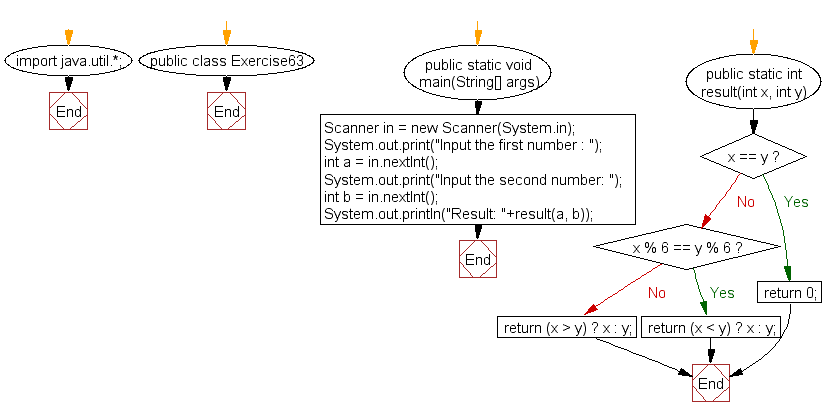﻿ Java: Accepts 2 integer and return the larger values# Java Exercises: Accepts two integer values from the user and return the larger values

## Java Basic: Exercise-63 with Solution

Write a Java program that accepts two integer values from the user and return the larger values. However if the two values are the same, return 0 and return the smaller value if the two values have the same remainder when divided by 6.
Test Data:
Input the first number : 12
Input the second number: 13
Result: 13
Input the first number : 12
Input the second number: 12
Result: 0
Input the first number : 6
Input the second number: 18
Result: 6

Sample Solution:

Java Code:

``````import java.util.*;
public class Exercise63 {
public static void main(String[] args)
{
Scanner in = new Scanner(System.in);
System.out.print("Input the first number : ");
int a = in.nextInt();
System.out.print("Input the second number: ");
int b = in.nextInt();
System.out.println("Result: "+result(a, b));
}
public static int result(int x, int y)
{
if(x == y)
return 0;
if(x % 6 == y % 6)
return (x < y) ? x : y;
return (x > y) ? x : y;
}
}
```
```

Sample Output:

```Input the first number : 12
Input the second number: 13
Result: 13
```

Pictorial Presentation:Flowchart:Java Code Editor:

What is the difficulty level of this exercise?

Test your Programming skills with w3resource's quiz.

﻿

## Java: Tips of the Day

countOccurrences

Counts the occurrences of a value in an array.

Use Arrays.stream().filter().count() to count total number of values that equals the specified value.

```public static long countOccurrences(int[] numbers, int value) {
return Arrays.stream(numbers)
.filter(number -> number == value)
.count();
}
```

Ref: https://bit.ly/3kCAgLb